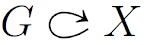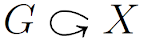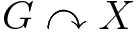# What is the standard notation for group action

Please let me know what is the standard notation for group action.

I saw the following three notations for group action. (All the images obtained as G\acts X for different deinitions of \acts.)

(1)I saw this one most, but only in handwriting and I like it. But I did not find a better way to write it in LaTeX.

\usepackage{mathabx,epsfig}
\def\acts{\mathrel{\reflectbox{$\righttoleftarrow$}}}


(2)It is almost as good as 1, but in handwriting this arrow can be taken as $G$.

\usepackage{mathabx}
\def\acts{\lefttorightarrow}


(3)I saw this one in print, I guess it is used since there is no better symbol in "amssymb".

\usepackage{amssymb}
\def\acts{\curvearrowright}

• There is no standard notation. Were I named Emperor of Notation, I would dictate a 90-degree rotated variant of your third option... May 10, 2011 at 21:37
• I'm pretty sure that the guys over at tex.stackexchange.com would have a LaTeX fix for what you want. May 10, 2011 at 22:00
• Whats wrong with "Let G act on X"? May 10, 2011 at 22:38
• I am not a native english speaker and only recently, I learned the term "bikeshedding" (see urbandictionary.com/define.php?term=bikeshedding), which is pretty much what this question is about. May 11, 2011 at 15:25
• @JackHuizenga: Nothing wrong with it, but if I write $f : X \to Y$ will you ask me what's wrong with "Let $f$ be a function from $X$ to $Y$"? Sep 2, 2014 at 22:46

I guess I am somewhat of a minimalist when it comes to notation. I have spent a lot of my career writing about group actions, and what I usually have done is start out defining what a group action is, and then say something like If we have in mind a fixed action of G on X then we will say that X is a G-space and we will denote by gx the result of the action of g in G on an element x of X.''

To be more pedantic, the point is that for a fixed group G, G-spaces form a category and if you ever had two different actions of G on the same topological space, you really should use two different symbols to denote that space with the different actions And it is convenient to have a morphism (equivariant map) $\phi$ between G-spaces just mean that $\phi(gx) = g \phi(x)$.

As it happens, I can still remember being confused the first few times I saw this notation: not to put too fine a point on it, but there is something syntactically new going on there beyond the usual function / arrow notation.

In my opinion this is not notation at all but rather shorthand. In other words, it is something that you can use in your own handwritten notes and something that you can write on the board if you are confident that your listeners will understand you. (I suppose if I start telling stories about all the talks I witnessed as a Harvard graduate student that sailed over my head whether the speaker intended them to or not, I will not get enough "Oh, you poor dear" responses to justify the effort. But it happened quite a lot!) It's nice to have an agreed upon shorthand. For instance, in the commutative algebra class I just taught, when things were hot and heavy I didn't want to keep writing out "$I$ is an ideal of $R$", so I used a shorthand for it and explained it the first 20 times I used it. (The students used it too when presenting solutions to problems.) But in my lecture notes it appears nowhere: if I have the time to tex up lecture notes at all, then I certainly have the time to write out "$I$ is an ideal of $R$".

Thus I would not recommend that anyone include this notation in their formal mathematical writings. Note that Dick Palais has said essentially the same thing above, so: don't listen to me, but listen to him!

• This basically expands upon the comment I left to the question, and I fully agree. May 11, 2011 at 11:24
• It is a shorthand, quite similar to the shorthand $X \to Y$ for a function. I think of them both as "unnamed" things. You can easily name the action using the same notation, sometimes I use something like $f : G \acts X$. Sep 2, 2014 at 22:49

As this was the first hit on google for "latex action arrow", but didn't contain what I wanted, let me post what I figured out. But to address other people's issues with the original question: while I agree that in a sentence one should simply say "Let $G$ act on $X$...", I was interested in drawing a (what some people just generically call "commutative") diagram in order to visually display the relationships between several objects (as you do).

Anyway, there is a symbol $\circlearrowright$, which is almost what I wanted. If you do this:

\usepackage{graphicx}
G\ \rotatebox[origin=c]{-90}{\$\circlearrowright\$}\ X

you will get an arrow whose beginning and end are right next to the X.

It is always a shame, of course, when none of the many LaTeX packages has precisely the symbol that you might use on the chalkboard. In writing, you should try to use words when possible, or at least supplement your symbols with words. Someone just reading the words should be able to pick up the nuances of your notation. Very importantly, use your notation consistently throughout the paper.

When speaking at the chalkboard, you should speak everything you write, and write everything you speak, in the following sense: someone blind should be able to follow the majority of your talk, and also someone deaf should be able to follow the majority of the talk. Even when talking one-on-one with someone else, this is important. So you write "$G \acts X$" for your favorite definition of $\acts$, but you speak "Let $G$ act on $X$." And in the most important statements in the talk, namely the statements of definitions and theorems, you should write all the words: "Let $G$ act on $X$."

Personally, I like some variation on your option 1. I have seen 3, and I use it in some lecture notes. I do not like 2: when people read, they tend to give a lot more visual importance to the tops of words and letters than to the bottoms (this is why almost all letters do not hang below the baseline, but there are many that stick up higher, and you can almost read just from knowing which letters go higher up). So put the arrow at the top.

• Allen Knutson once pointed out to me that you should always use (1) instead of (2) because in many people's handwriting, the arrow looks a lot like a $G$. May 10, 2011 at 23:17
• How about $\alpha:G\timesX\rightarrow X$, with $\alpha(g,x)= g\star x$? Of course, I would prefer $\alpha(g,x)= g \cdot x$, and an articulation: $1\cdot x=x$, (gh)\cdot x= g \cdot(h\cdot x)$. Similar notation holds for right actions. May 10, 2011 at 23:48 • Scott, that gives you a notation for the action itself, but the proposed notations mean «$G$acts on$X$», and just expresses the fact that there is an action. May 10, 2011 at 23:55 • @Ben: OP makes a similar point. @Scott: There are situations where I have a reasonable notion of "acts on", which is more complicated than a map$G \times X \to X$; e.g. if$G$is a (Hopf) algebra and$X$a representation, then I certainly don't mean categorical$\times$. But more generally it would be better to write$G \to \operatorname{End}(X)$than$G \times X \to X$, because then it at least reminds one that the map should be a homomorphism of something. In some settings, though, I think it is not always known what the correct definition of "$\operatorname{End}$" is, e.g.$E_n$algebras. May 11, 2011 at 1:42 • @Harry: A topological group is not (just) a group, and a group scheme is definitely not a group. So your examples lie outside of my philosophy. Perhaps we could talk about "enriched categories"...but I'd rather not. May 11, 2011 at 6:50 IN addition to what has been said, I would like to mention the following aspect: a group action usually does not come alone. When$G$acts on a set$X$you will almost for sure be interested also in the induced action of$G$on certain kind of functions on$X$: think of a smooth manifold$M$and of smooth functions, or of smooth tensor fields on$M$, or what not. Then the induced group action can be either viewed as a right action when$G$acts from the left on$X$or you have to plug in a$^{-1}$to turn things again into a left action. In various situations (say equivariant maps in the dual of a Lie algebra etc) it might become a notational desaster if you want to specify all kind of actions with a separate symbol. However, it might be important to keep track of whether you have a left or a right action. So my habit is to denote the action of a group element$g$on some object$x \in X$by$g \triangleright x$if it is a left action and by$g \triangleleft x$or better$x \triangleleft g$if it is a right action. Then you don't have to bother whether you have already included the$^{-1}\$ in the coadjoint action to make it a left action or just stay with a right action :)